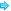科研进展新闻动态科研进展综合新闻传媒扫描
 现在位置：首页 > 新闻动态 > 科研进展

2023-09-20 | 编辑：

The Modular Inversion Hidden Number Problem (MIHNP), which was proposed at Asiacrypt 2001 by Boneh, Halevi, and Howgrave-Graham, is summarized as follows: Assume that the $\delta$ most significant bits of $z$ are denoted by }_{\delta }(z)$. The goal is to retrieve the hidden number$\alpha \in \mathbb {Z}_{p}$given many samples$\left ({t_{i}, {\mathrm {MSB}}_{\delta }((\alpha + t_{i})^{-1} \bmod {p})}\right)$for random$t_{i} \in \mathbb {Z}_{p}$. MIHNP is a significant subset of Hidden Number Problems. Eichenauer and Lehn introduced the Inversive Congruential Generator (ICG) in 1986. It is basically characterized as follows: For iterated relations$v_{i+1}=(av^{-1}_{i}+b)\bmod {p}$with a secret seed$v_{0} \in \mathbb {Z}_{p}$, each iteration produces$\mathrm {MSB}_{\delta }(v_{i+1})$where$i \geq 0$. The ICG family of pseudorandom number generators is a significant subclass of number-theoretic pseudorandom number generators. Sakai-Kasahara scheme is an identity-based encryption (IBE) system proposed by Sakai and Kasahara. It is one of the few commercially implemented identity-based encryption schemes. We explore the Coppersmith approach for solving a class of modular polynomial equations, which is derived from the recovery issue for the hidden number$\alpha $in MIHNP and the secret seed$v_{0}$in ICG, respectively. Take a positive integer$n=d^{3+o(1)}$for some positive integer constant$d$. We propose a heuristic technique for recovering the hidden number$\alpha $or secret seed$v_{0}$with a probability close to 1 when$\delta /\log _{2} p>\frac {1}{d+1}+o\left({\frac {1}{d}}\right)$. The attack’s total time complexity is polynomial in the order of$\log _{2} p$, with the complexity of the LLL algorithm increasing as$d^{\mathcal {O}(d)}$and the complexity of the Gr？bner basis computation increasing as$d^{\mathcal {O}(n)}$. When$d> 2$, this asymptotic bound surpasses the asymptotic bound$\delta /\log _{2} p>\frac {1}{3}$established by Boneh, Halevi, and Howgrave-Graham at Asiacrypt 2001. This is the first time a more precise constraint for solving MIHNP is established, implying that the claim that MIHNP is difficult is violated whenever$\delta /\log _{2} p < \frac {1}{3}\$ . Then we study ICG. To our knowledge, we achieve the best performance for attacking ICG to date. Finally, we provide an MIHNP-based lattice approach that recovers the signer’s secret key in the Sakai-Kasahara type signatures when the most (least) significant bits of the signing exponents are exposed. This improves the existing work in this direction.

Publication:

IEEE Transactions on Information Theory, vol. 69, no. 8, pp. 5337-5356, Aug. 2023

Author:

Jun Xu

State Key Laboratory of Information Security, Institute of Information Engineering, Chinese Academy of Sciences, Beijing, China

School of Cyber Security, University of Chinese Academy of Sciences, Beijing, China

Santanu Sarkar

Indian Institute of Technology Madras, Chennai, India

Lei Hu

State Key Laboratory of Information Security, Institute of Information Engineering, Chinese Academy of Sciences, Beijing, China

School of Cyber Security, University of Chinese Academy of Sciences, Beijing, China

Huaxiong Wang

Division of Mathematical Sciences, School of Physical and Mathematical Sciences, Nanyang Technological University, Jurong West, Singapore

Yanbin Pan

Key Laboratory of Mathematics Mechanization, NCMIS, Academy of Mathematics and Systems Science, Chinese Academy of Sciences, Beijing, China

Email: panyanbin@amss.ac.cn

【打印本页】【关闭本页】